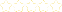• > Algebra II-Chapter 5
+# Algebra II-Chapter 5

##### Description:
This collection includes all lessons from Chapter 5.

### Your Quiz Points in this Playlist

Total Possible
0
9 Tutorials in this Playlist

#### Algebra II Lesson 5.3 "Transforming Parabolas (Vertex Form)"

Rating:(2)

#### Algebra II Lesson 5.3 "Transforming Parabolas (Writing Quadratic Models)"

Rating:(6)

#### Algebra II Lesson 5.2 "Properties of Parabolas" (Standard Form)

Rating:(8)

#### Algebra II "Intercepts Form of Quadratic Functions"

Rating:(1)

#### Algebra II "Writing Quadratic Functions in Intercepts Form"

Rating:(15)

#### Algebra II Lesson 5.4 & 5.5 "Factoring Quadratic Expressions and Quadratic Equations"

Rating:(9)

#### Algebra II Lesson 5.5 & 5.8 "Solving Quadratic Equations by Graphing and The Quadratic Formula"

Rating:(2)

#### Algebra II Lesson 5.6 "Imaginary Numbers"